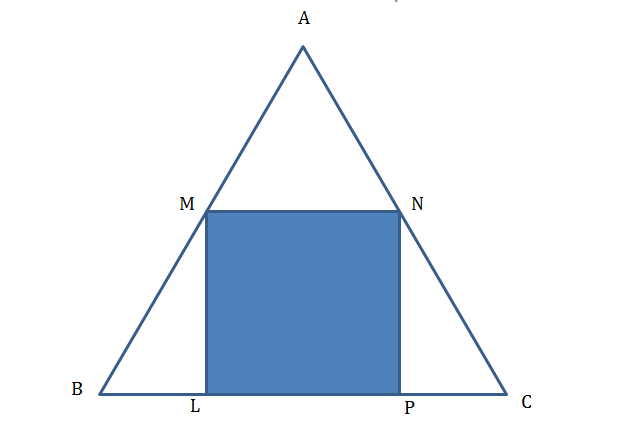# CAT Quantitative Aptitude Questions | Geometry Questions for CAT - Triangles

###### CAT Questions | CAT Geometry Questions | Triangle and Square

The question is from CAT Geometry - Triangles. It discusses about an equilateral triangle with a square inscribed inside it. We have to find out the ratio of the area of the square to that of the equilateral triangle. CAT Geometry questions are heavily tested in CAT exam. Make sure you master Geometry problems.

Question 25: There is an equilateral triangle with a square inscribed inside it. One of the sides of the square lies on a side of the equilateral △. What is the ratio of the area of the square to that of the equilateral triangle?

1. √3 : (5 + 4√3)
2. 2√3 : (7 + 4√3)
3. 4√3 : (7 + 4√3)
4. 4√3 : (5 + 2√3)

## Best CAT Coaching in Chennai

#### CAT Coaching in Chennai - CAT 2021Online Batches Available Now!

##### Method of solving this CAT Question from Triangles: Find the ratio of the side of the square and the side of the triangle and you are through.

Let side of square be ‘a’
MN = LP = a
∠ABC = 60°
ML = a. BL = $$frac{a}{√3}\\$$Use some trigonometry for this)PC = $$frac{a}{√3}\\$ Bc = a + $\frac{2a}{√3}\\$We are done Area of square = a2 Area of equilateral triangle = $\frac{√3}{4}\\$ *$a + $$frac{2a}{√3}\\$) 2 = $\frac{√3}{4*3}\\$ * a2$√3 + 2)2
= $$frac{1}{4*√3}\\$ * a2$7 + 4√3)
Ratio = 4√3 : (7 + 4√3)

The question is "What is the ratio of the area of the square to that of the equilateral triangle?"

##### Hence, the answer is 4√3 : (7 + 4√3)

Choice C is the correct answer.

###### CAT Coaching in ChennaiCAT 2021Enroll at 49,000/- 44,000/-

Online Classroom Batches Starting Now!

###### Best CAT Coaching in ChennaiPrices slashed by Rs 4000/-

Attend a Demo Class

## CAT Preparation Online | CAT Geometry questions Videos On YouTube

#### Other useful sources for Geometry Question | Geometry Triangles Circles Quadrilaterals Sample Questions

##### Where is 2IIM located?

2IIM Online CAT Coaching
A Fermat Education Initiative,
58/16, Indira Gandhi Street,
Kaveri Rangan Nagar, Saligramam, Chennai 600 093

##### How to reach 2IIM?

Phone: (91) 44 4505 8484
Mobile: (91) 99626 48484
WhatsApp: WhatsApp Now
Email: prep@2iim.com